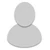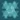## FANDOM

1,130 Pages# Sannse

Staff
• My occupation is Director of Community Support at Fandom.
• I am Back!
• Bio My dog is my obsession, making jewelry is my passion, chocolate is my addiction, and Fandom is my delight.• Hey Sannse,I decided to make a message for you but...have you checked the sploder site yet? =) If you are a sploder user then find me on my sploder profile page "goldenman99".If you don't,I recommend to look at the website,it's for kids and for adults too! :) You should check out the website...there it is! www.sploder.com

•• ) :) :) :) :) :) :) :) :) :) :) :) :) :) :) :) :) :) :) :) :) :) :) :) :) :) :) :) :) :) :) :) :) :) :) :) :) :) :) :) :) :) :) :) :) :) :) :) :) :) :) :) :) :) :) :) :) :) :) :) :) :) :) :) :) :) :) :) :) :) :) :) :) :) :) :) :) :)  :) :) :) :) :) :) :) :) :) :) :) :) :) :) :) :) :) :) :) :) :) :) :) :) :) :) :) :) :) :) :) :) :) :) :) :) :) :) :)  :) :) :) :) :) :) :) :) :) :) :) :) :) :) :) :) :) :) :) :) :) :) :) :) :) :) :) :) :) :) :) :) :) :) :) :) :) :) :)  :) :) :) :) :) :) :) :) :) :) :) :) :) :) :) :) :) :) :) :) :) :) :) :) :) :) :) :) :) :) :) :) :) :) :) :) :) :) :) :) :) :) :) :) :) :) :) :) :) :) :) :) :) :) :) :) :) :) :) :) :) :) :) :) :) :) :) :) :) :) :) :) :) :) :) :) :) :)  :) :) :) :) :) :) :) :) :) :) :) :) :) :) :) :) :) :) :) :) :) :) :) :) :) :) :) :) :) :) :) :) :) :) :) :) :) :) :) :) :) :) :) :) :) :) :) :) :) :) :) :) :) :) :) :) :) :) :) :) :) :) :) :) :) :) :) :) :) :) :) :) :) :) :) :) :) :) :) :) :) :) :) :) :) :) :) :) :) :) :) :) :) :) :) :) :) :) :) :) :) :) :) :) :) :) :) :) :) :) :) :)
• Thank you :)

• )
•• if you dont you should its a really fun site even for adults! if you have spare time check this out!  http://www.sploder.com/

•• The users "Pikajoey", "Greenkitty", and "Zorderys" are vandalizing my page I've worked hard on. Please stop them.Sorted out and to avoid notifying participating users. (Please start a new thread.)
00:48, September 17, 2014
• View all 6 replies
• Please can you block aqi0bsploder he keeps bullying me and won't leave me alone

• 10liamsl wrote:
Please can you block aqi0bsploder he keeps bullying me and won't leave me alone

Aqi0bsploder is not going to be blocked.

Closed.

• Hello Sannse, how do you add a category to any article on the sploder wiki.

• Thanks for the message

• 10liamsl wrote:
Thanks for the message

???

••